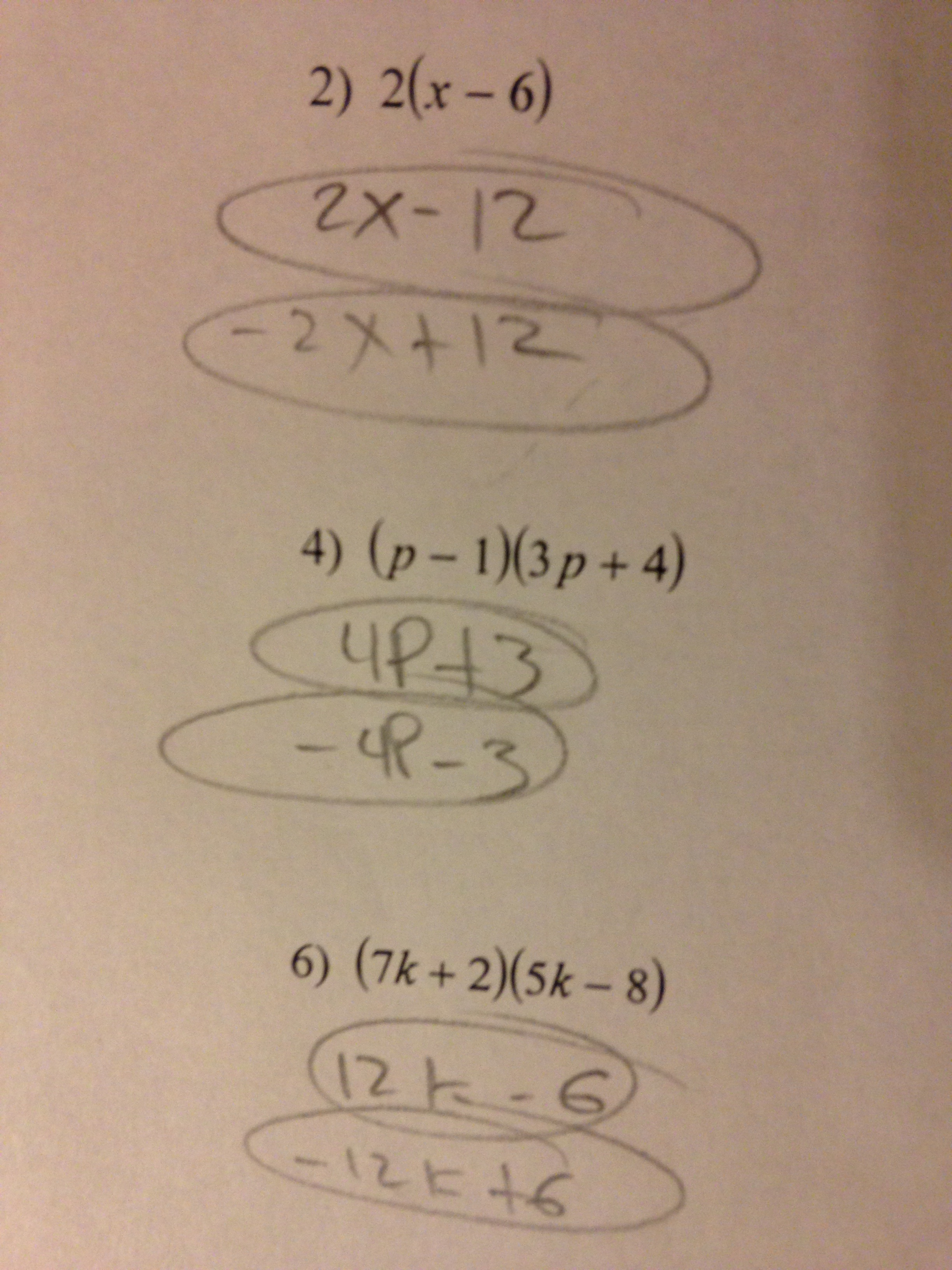Categories

# Multiplying monomials and binomialsThere’s a lot going on here. What about this work do you find the most interesting? How would you help this student?

Thanks to Heather for the submission!

## 2 replies on “Multiplying monomials and binomials”

The most interesting thing to me is the second answer where the student lists the additive inverse of each solution.

I wonder if the student has already learned to solve quadratic equations by factoring. That would influence my next step.

I’m still so puzzled by this one! Can anyone tell us more about what the student was thinking in giving the second answer? The first answer is clear enough, on the second and third problems confusing juxtaposition with addition when our (bad!) notation tells us that we’re supposed to confuse it with multiplication instead. Another chance for me to plug the idea that, through algebra 1, we ought to write multiplication dots when we mean multiplication!